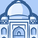# QlikView App Dev

Discussion Board for collaboration related to QlikView App Development.

Announcements
QlikWorld 2023, a live, in-person thrill ride. Save \$300 before February 6: REGISTER NOW!
cancel
Showing results for
Did you mean:Specialist II

## Logic to increase values each year based on a given date

Hi guys,

I have a table with the following data

ID     Amount     Increase Date     Increase PCT

1     1000          01/01/2016               3

2     1200          04/01/2015               2

3     1500          09/01/2015               2

I want to apply a logic to increase the amount by the PCT column once we pass the increase date.. which I have already completed..

the request now is that we need to re-increase the value (Increase PCT *2) next year when we hit the same date again and so on future years..

Any thoughts on how to best build the logic

Best,

Alec

1 Solution

Accepted SolutionsSpecialist II
Author

I got it figured out using the following logic..

if([Increase Date]>Today(),0,

if([Increase Date]<Today() and Year([Increase Date])=Year(Today()),1,

if([Increase Date]<Today() and Year([Increase Date])<Year(Today()) and num(month([Increase Date]))&num(Day([Increase Date])) <= num(month(Today()))& num(Day(Today())), Year(Today())-Year([Increase Date])+1,

if([Increase Date]<Today() and Year([Increase Date])<Year(Today()) and num(month([Increase Date]))&num(Day([Increase Date]))>   num(month(Today()))& num(Day(Today())),(Year(Today())-Year([Increase Date])))))) as IncreaseFactor

Thank you!

6 RepliesMVP

What is the Date with whom you want to compare?

May be like this?

The logic used below is

If Date > Today, Amount * 1,

If Date > Today and Difference is More than Year, Amount * 2.......

etc

etc

====================================

Table:

*,

Amount * If(Flag = 'Y', Year(Today()) - Year([Increase Date])+1) as NewAmount;

ID,

Amount,

[Increase Date],

[Increase PCT],

If([Increase Date] < Today(), 'Y','N') as Flag

Inline

[

ID,   Amount,     Increase Date,     Increase PCT

1,    1000,          10/03/2012,               3

2,    1200,          10/03/2013,               2

3,    1500,          10/03/2014,               2

4,    1800,          10/03/2015,               2

5,    2000,          20/03/2015,               2

];Specialist II
Author

thank you for the help but I dont think the logic calculate correctly.. for example the first line has increase PCT of 3. and increase date 10/3/2012.. we have passed that date so the amount should be increased by 3% on each year we passed that date which should result to 12% (3 % each year from the date of increase)..MVP

Provide another sample data alongwith the expected output..

or use

Amount * (If(Flag = 'Y', Year(Today()) - Year([Increase Date])+1)/100) as NewAmount;Specialist II
Author

Here is the above data along with the output increase PCT.. once we know that we can multiply with the amount

ID,   Amount,     Increase Date,     Increase PCT   Expected PCT

1,    1000,          10/03/2012,               3                    12

2,    1200,          10/03/2013,               2                    6

3,    1500,          10/03/2014,               2                    4

4,    1800,          10/03/2015,               2                    2

5,    2000,          20/03/2015,               2                    0MVP

Which date is the comparing date?Specialist II
Author

I got it figured out using the following logic..

if([Increase Date]>Today(),0,

if([Increase Date]<Today() and Year([Increase Date])=Year(Today()),1,

if([Increase Date]<Today() and Year([Increase Date])<Year(Today()) and num(month([Increase Date]))&num(Day([Increase Date])) <= num(month(Today()))& num(Day(Today())), Year(Today())-Year([Increase Date])+1,

if([Increase Date]<Today() and Year([Increase Date])<Year(Today()) and num(month([Increase Date]))&num(Day([Increase Date]))>   num(month(Today()))& num(Day(Today())),(Year(Today())-Year([Increase Date])))))) as IncreaseFactor

Thank you!Community Browser